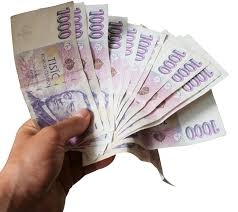# Three-fifths 7447

Silvia had a certain amount of money in her wallet. She spent a third of it on stationery and spent three-fifths of the remaining money on groceries. She has €76 left. How much € did Silvia initially have in her wallet?

x =  285 Eur

### Step-by-step explanation:

x - x/3 - 3/5·(x - x/3) = 76

4x = 1140

x = 1140/4 = 285

x = 285

Our simple equation calculator calculates it.Did you find an error or inaccuracy? Feel free to write us. Thank you!

Tips for related online calculators
Need help calculating sum, simplifying, or multiplying fractions? Try our fraction calculator.
Check out our ratio calculator.
Do you have a linear equation or system of equations and looking for its solution? Or do you have a quadratic equation?